5709

Mathematics Integration Level: Misc Level

Prove that the curves xy - 4 and x2 + y2 - 8 touch each other.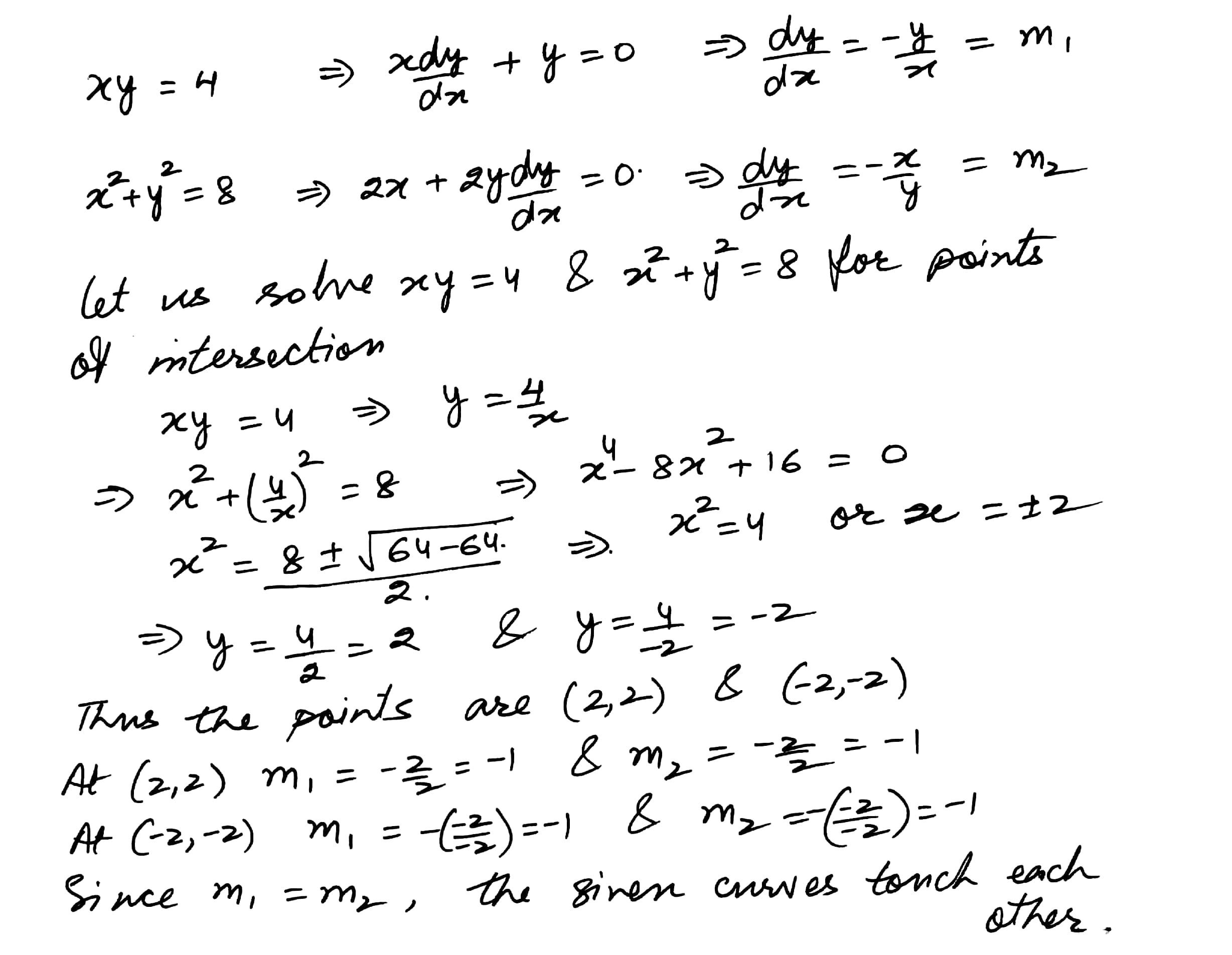5708

Mathematics Integration Level: Misc Level

Prove that the curves y = x2 - 3x + 1 and x(y + 3) - 4 intersect at right angles at the poin (2, -1).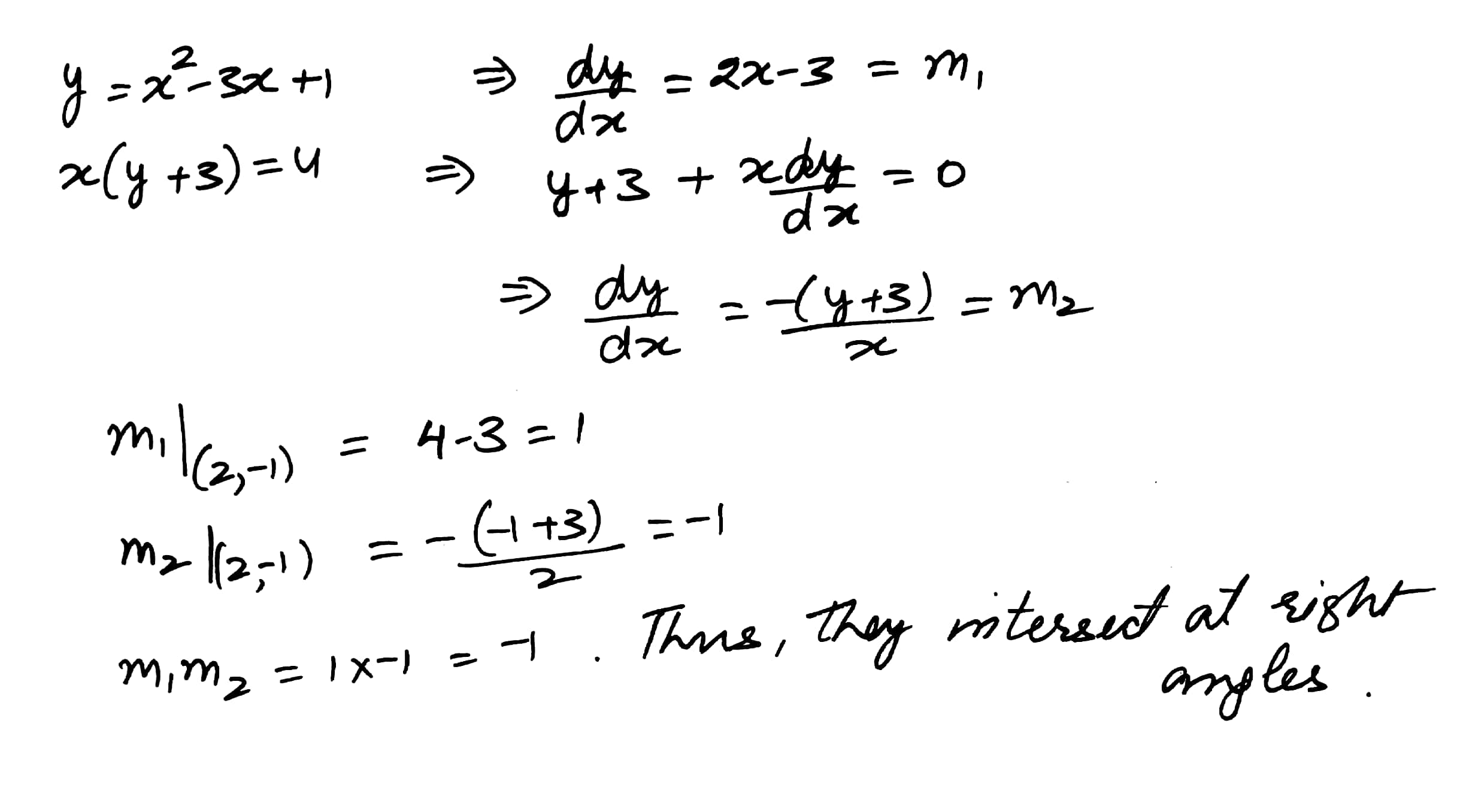5707

Mathematics Integration Level: Misc Level

Find the equations of the tangents to the curve y = x3 + 2x - 4 which are perpendicular to theline x + 14y + 3 = 0.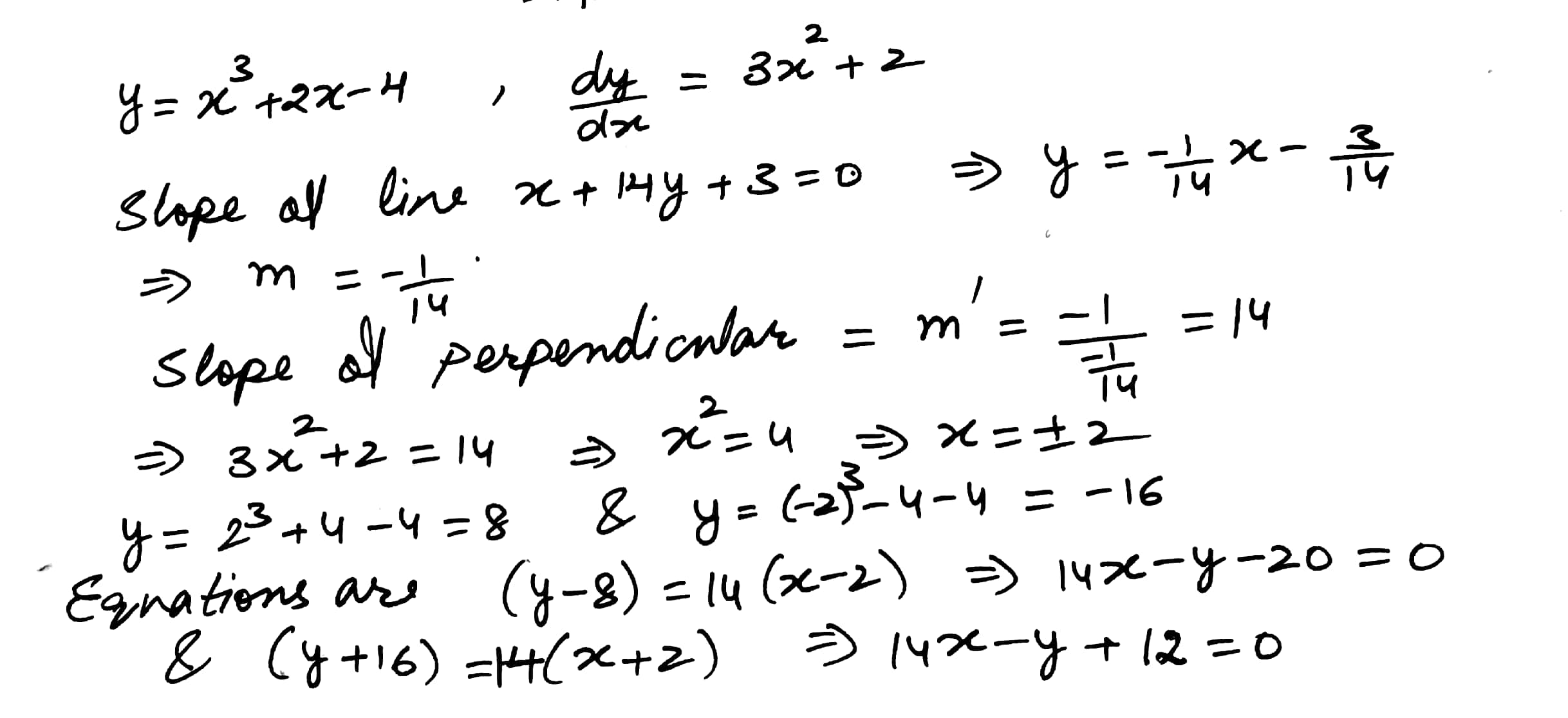5706

Mathematics Integration Level: Misc Level

Find the equations of all lines having slope 2 and that are tangents to the curve .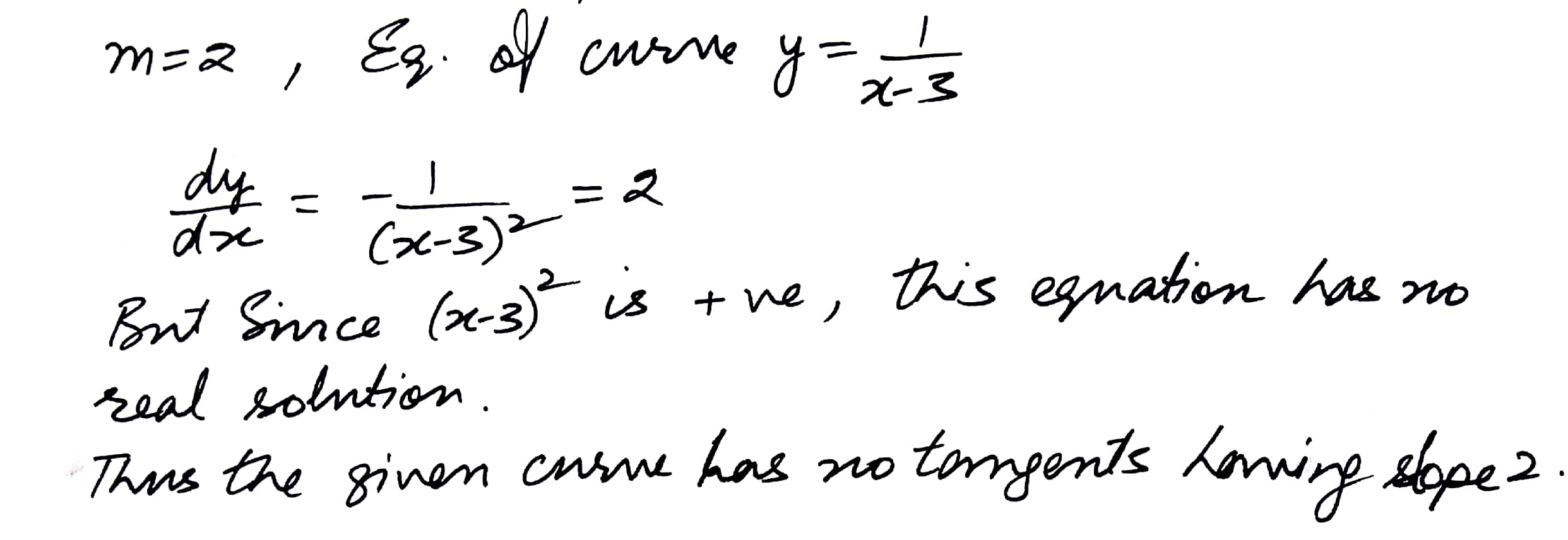5705

Mathematics Integration Level: Misc Level

Find the equations of the tangent to the curve x = Sin 3t, y = Cos 2t at .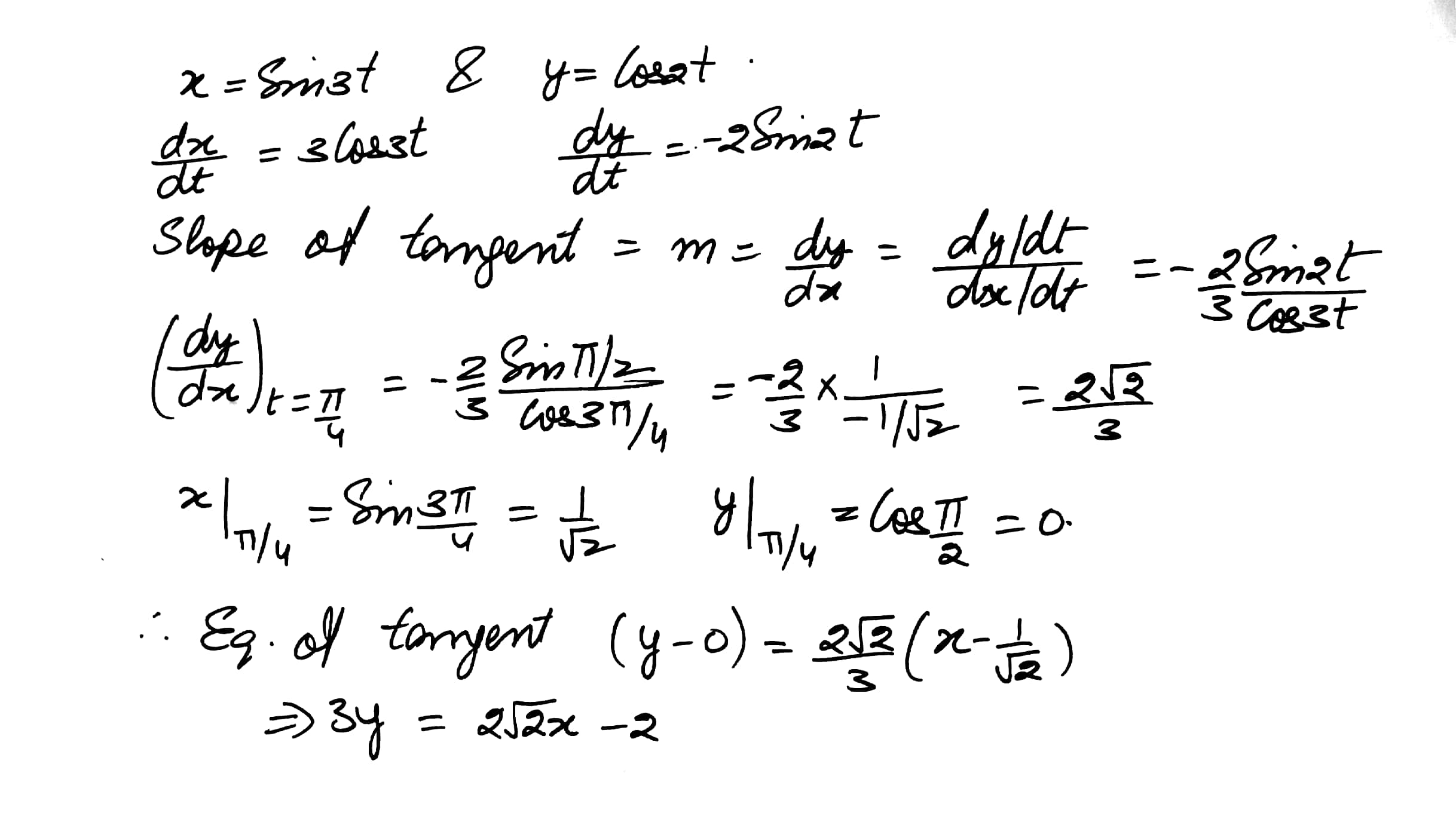5704

Mathematics Integration Level: Misc Level

Find the equations of the tangent and the normal to the curve   at (1,1).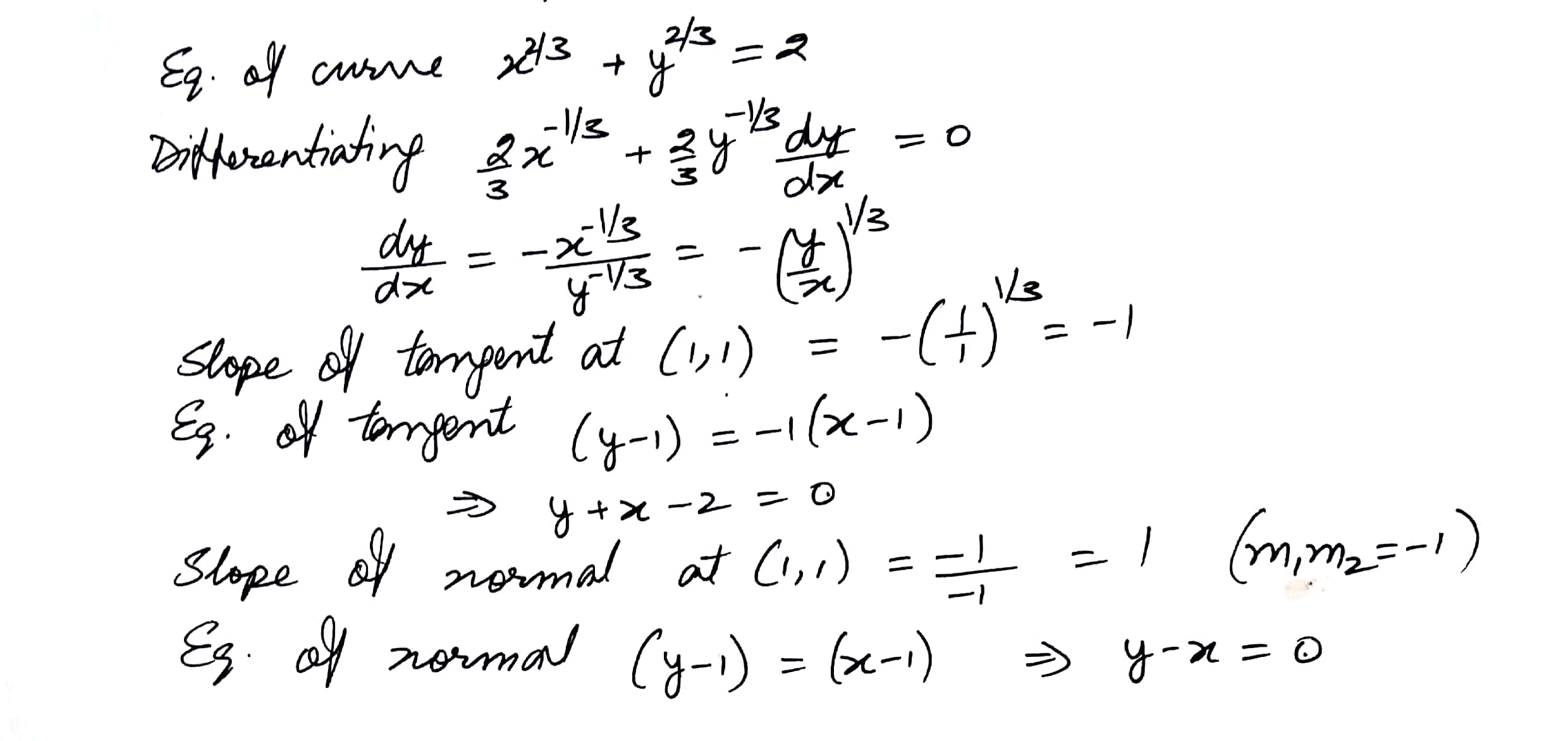5703

Mathematics Integration Level: Misc Level

Find the equations of the tangent and the normal to the curve y = x4 - 6x3+13x2 - 10x + 5 at (1,3).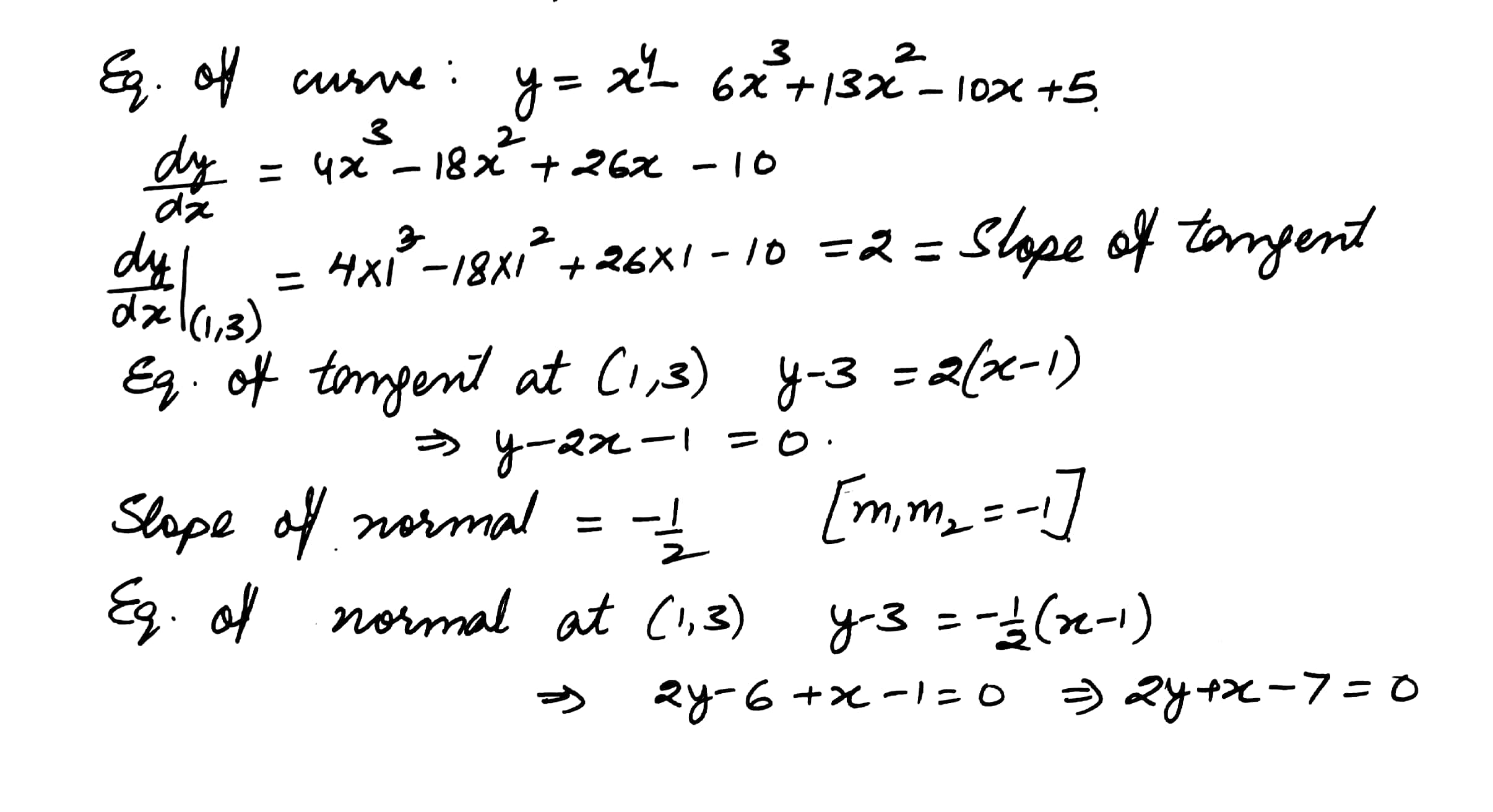5702

Mathematics Integration Level: Misc Level

Find the points on the curve at which the tangents are.

(a)  parallel to x-axis

(b)  parallel to y-axis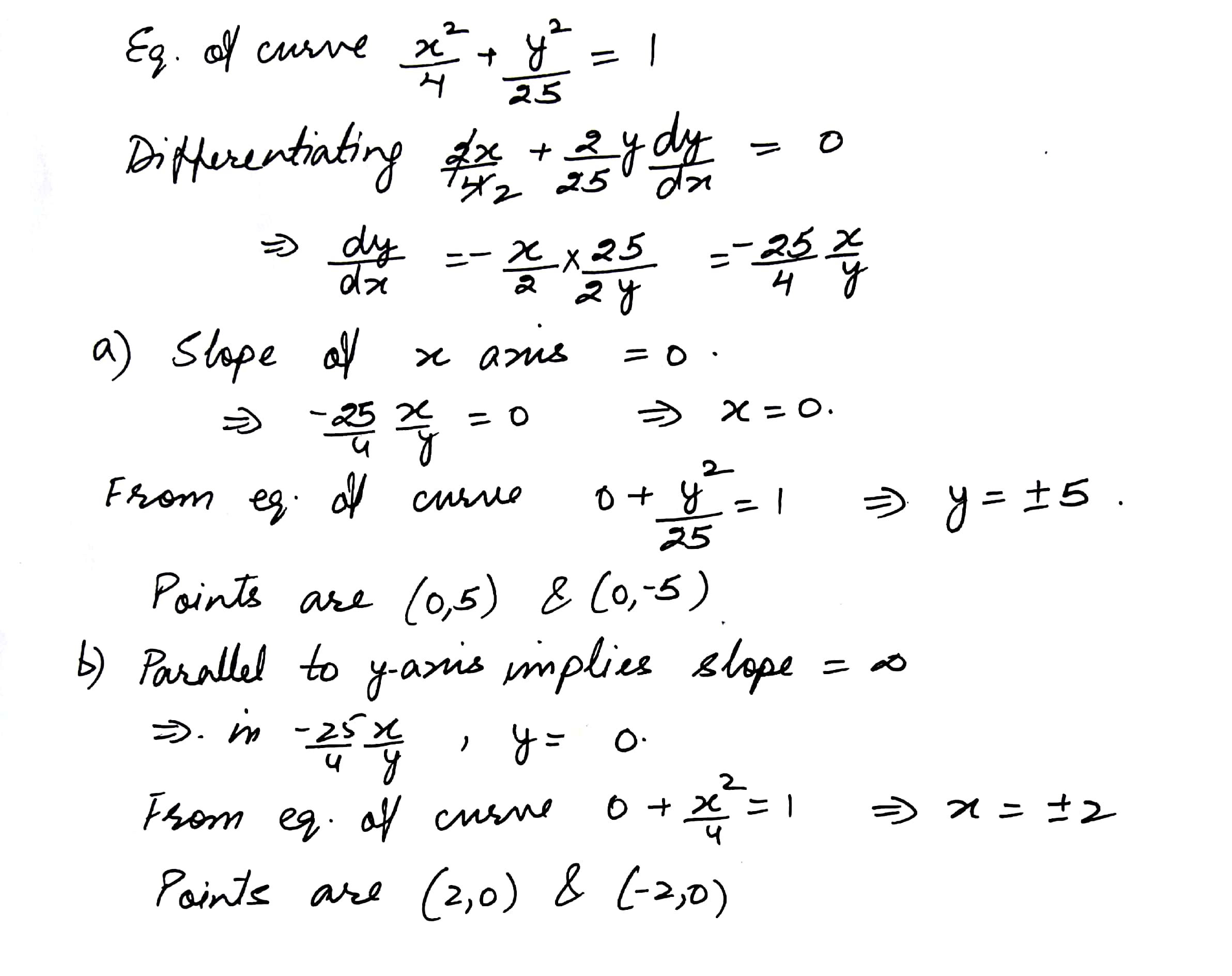5701

Mathematics Integration Level: Misc Level

Determine the point of the curve y=3x2-5 at which the tangent is perpendicular to the line whose slope is -1/3.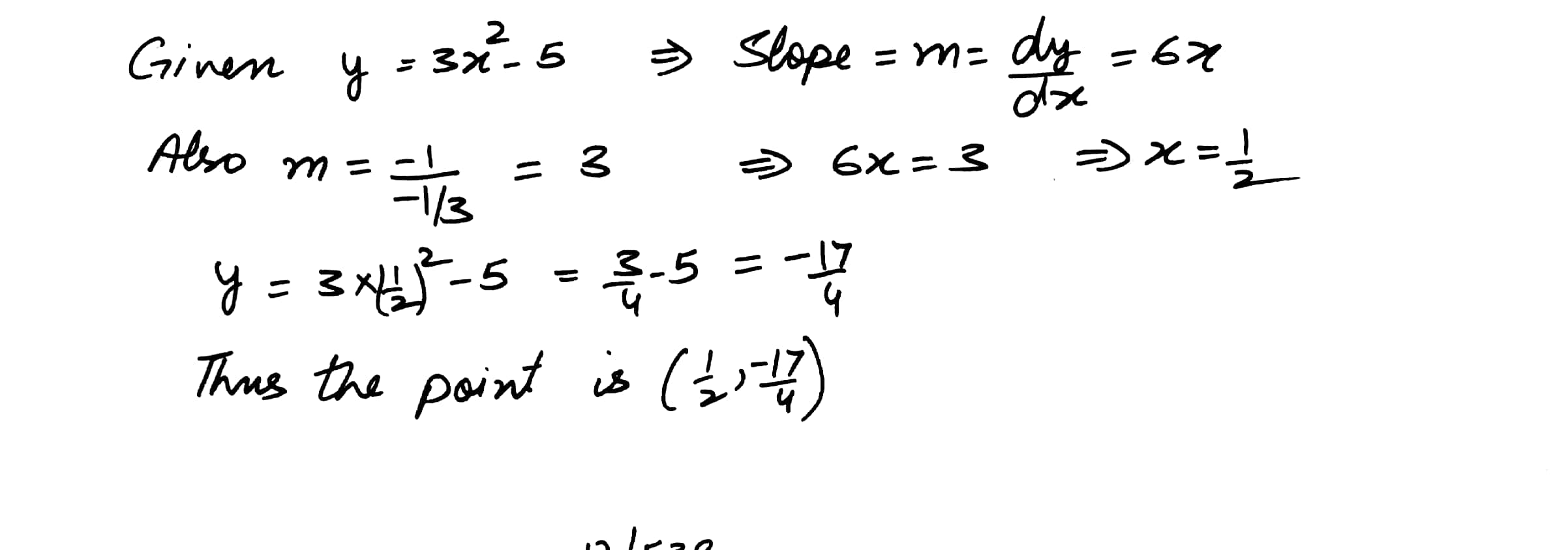5700

Mathematics Integration Level: Misc Level

If the tangent to the curve y=x3++ax+b at (1,-6) is parallel to the line 2x-2y+7=0, find a anb b.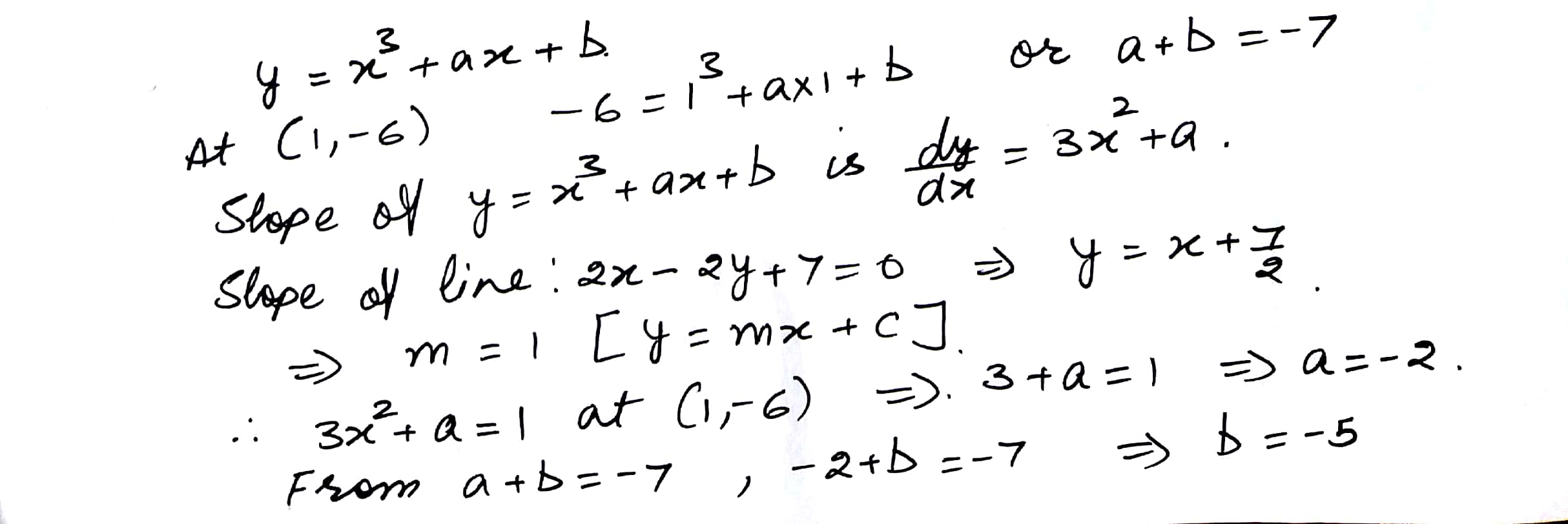5699

Mathematics Integration Level: Misc Level

Show that the tangents to the curve at the points where x=2 and x=-2 are parallel.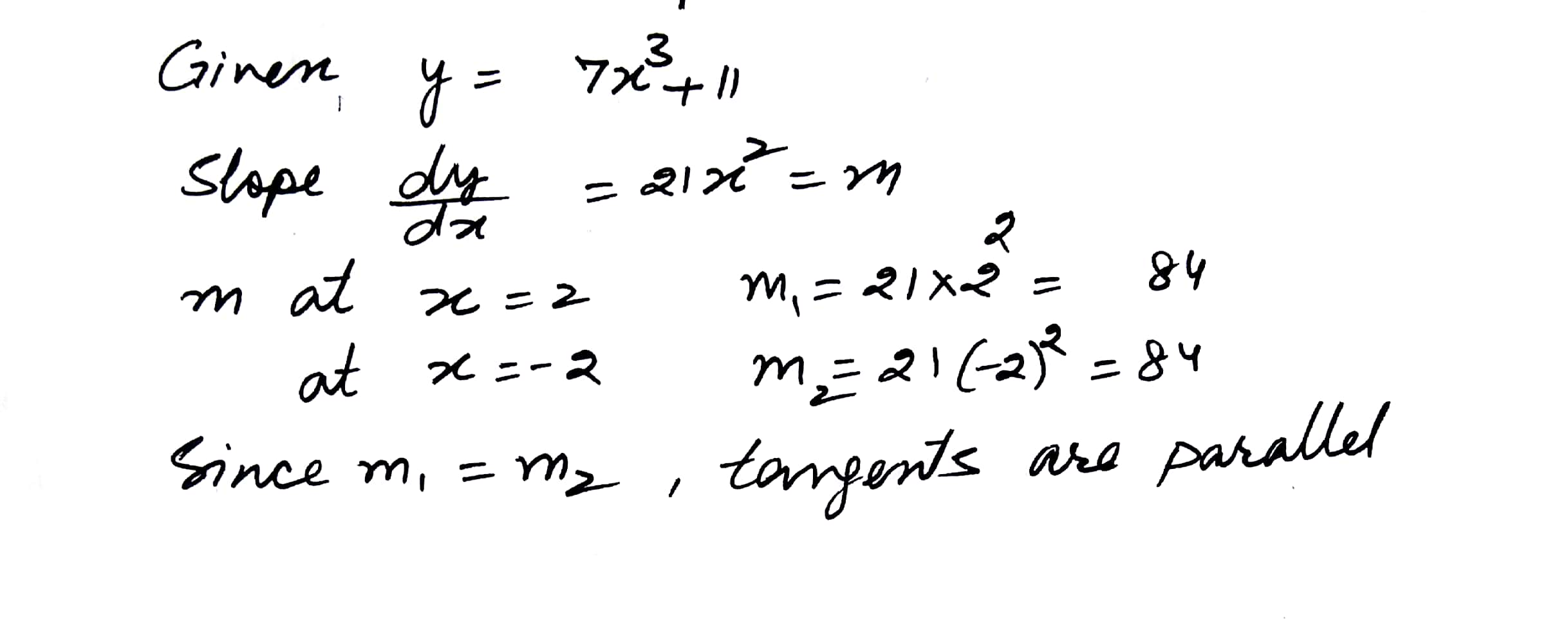5698

Mathematics Integration Level: Misc Level

Find the slopes of the tangents at the three points where the curve cuts the x-axis.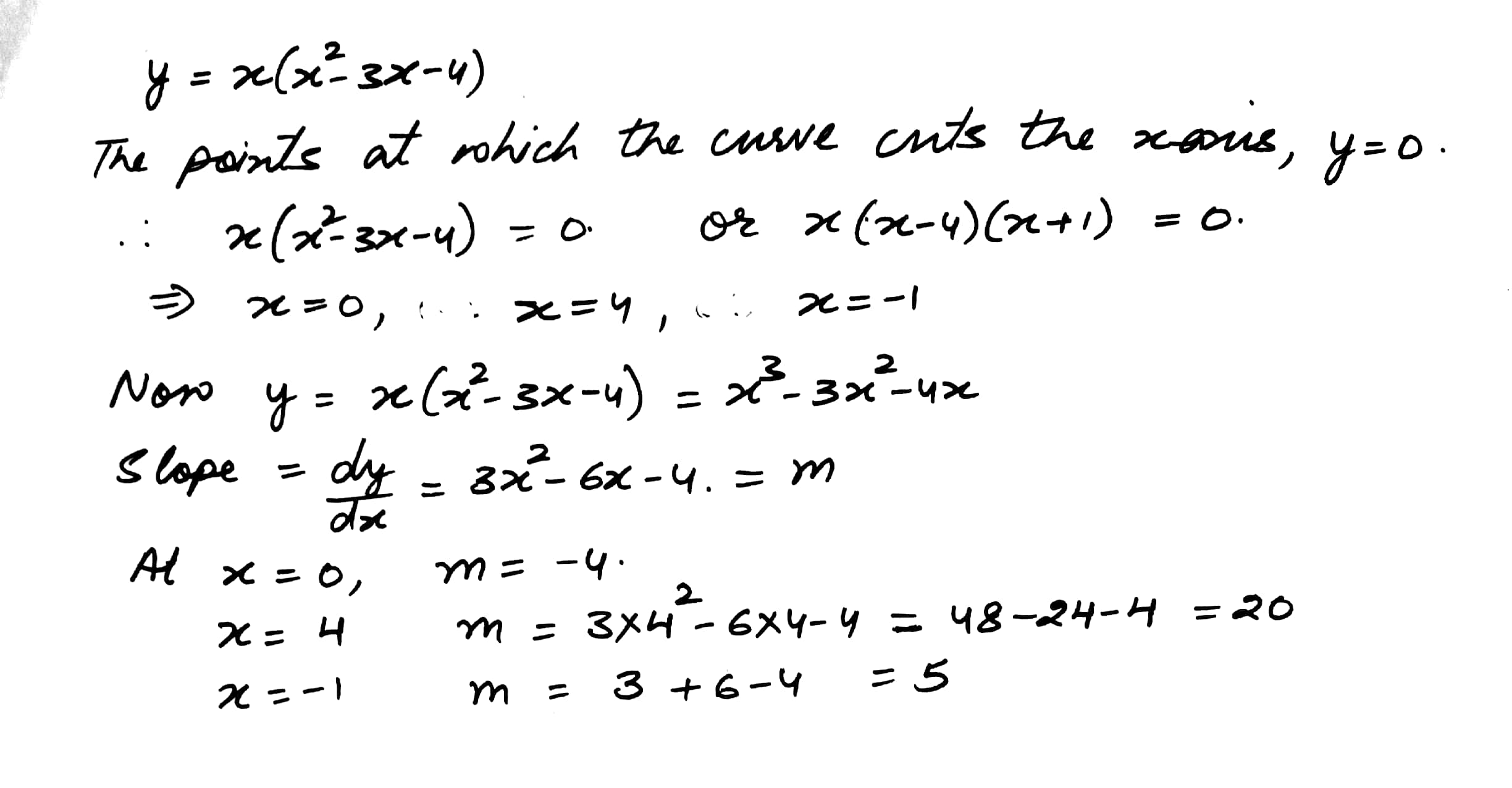5567

Mathematics Integration Level: Misc Level

p(x)=0.03x3+0.2x2+15x+100 represents the air pollution in an industrial area due to smoke produced by x chimneys. Find the marginal value of air pollution when 3 chimneys are increased. Which value does this question indicate?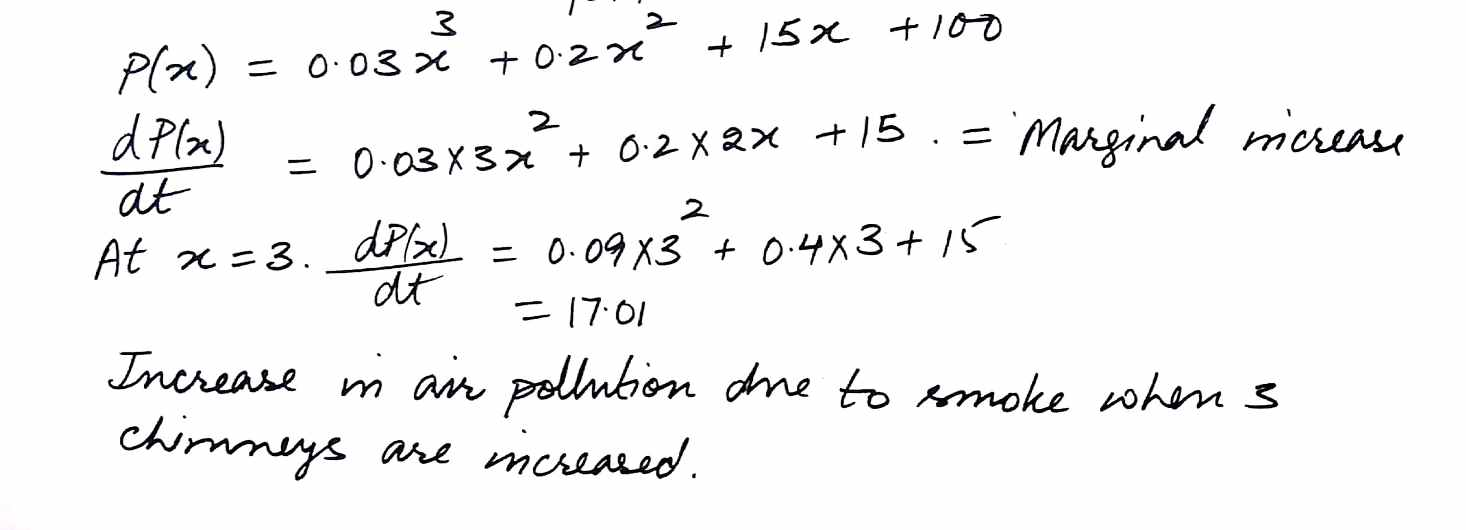5566

Mathematics Integration Level: Misc Level

Water is dripping out at a steady rate of 1 cu cm/sec through a tiny hole at the vertex of the conical vessel, whose axis is vertical. When the slant height of water in the vessel is 4 cm, find the rate of decrease of slant height, where the semi-vertical angle of the cone is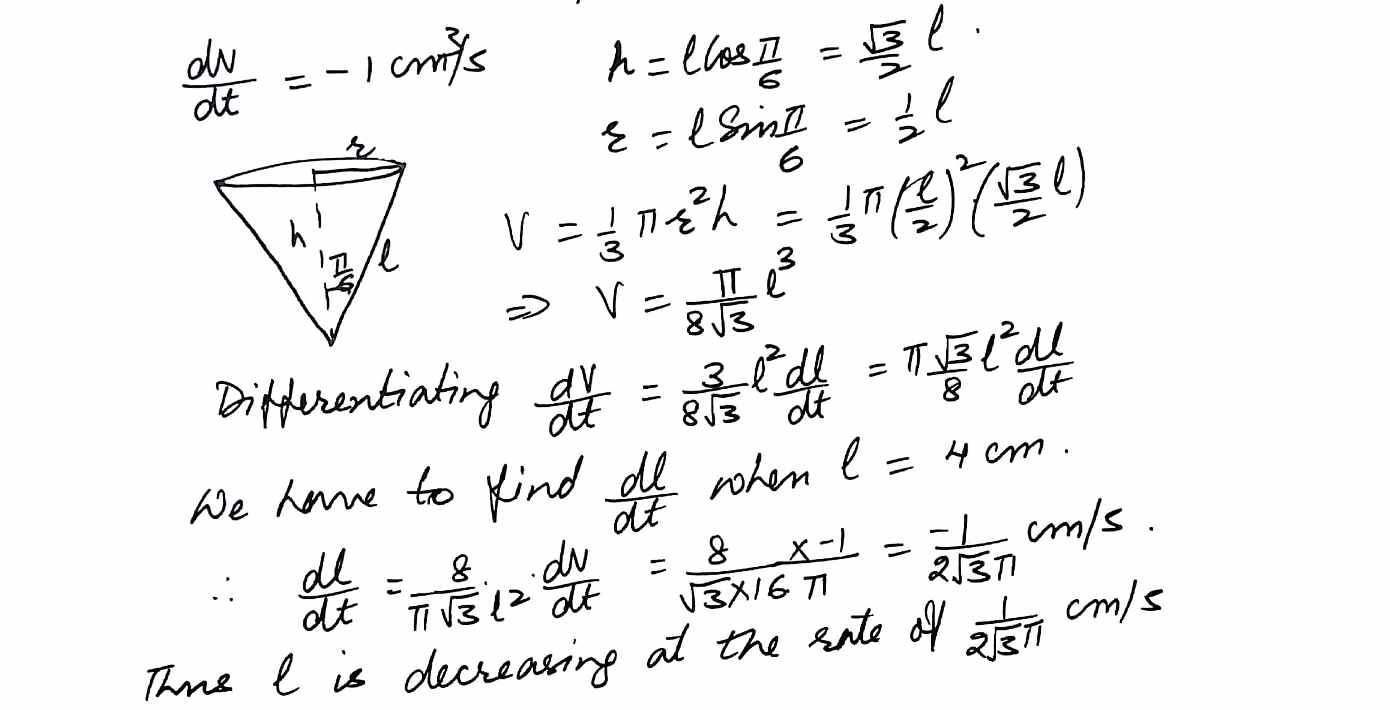5565

Mathematics Integration Level: Misc Level

A conical vessel whose height is 4 meters and the base radius 2 meters is being filled with water at the rate of 0.75 cubic meters per minute. Find the rate at which the level of the water is rising when the depth of water is 1.5 meters.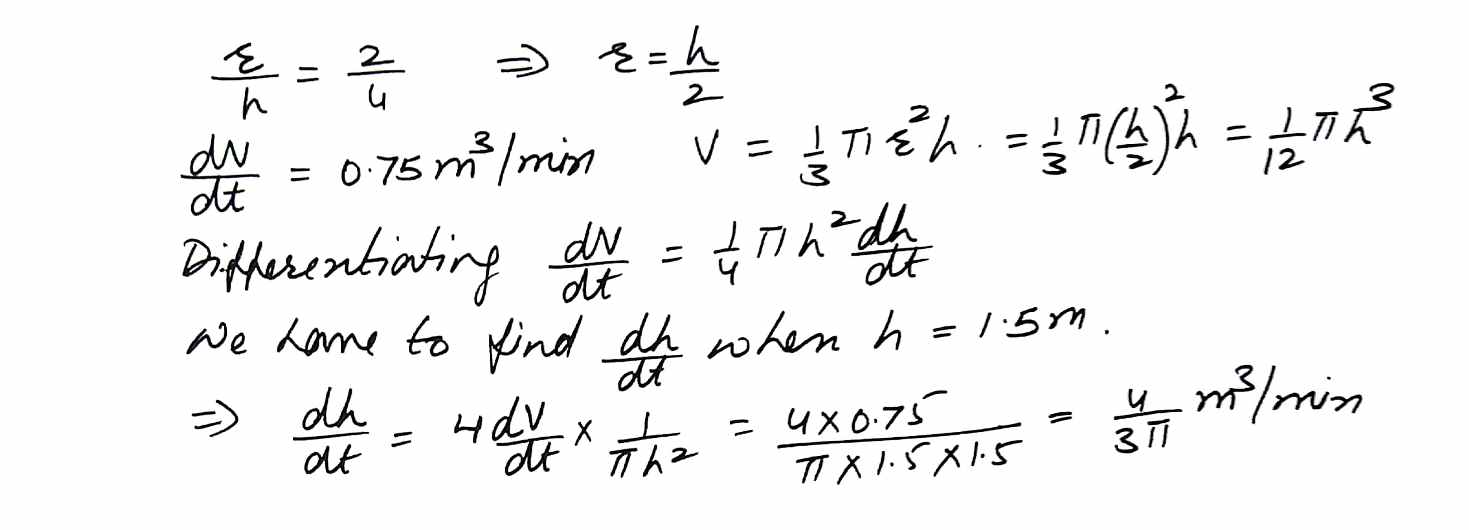Displaying 46-60 of 195 results.